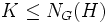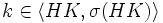# Intermediate isomorph-conjugacy is normalizing join-closed

This article gives the statement, and possibly proof, of a subgroup property (i.e., intermediately isomorph-conjugate subgroup) satisfying a subgroup metaproperty (i.e., normalizing join-closed subgroup property)
View all subgroup metaproperty satisfactions | View all subgroup metaproperty dissatisfactions |Get help on looking up metaproperty (dis)satisfactions for subgroup properties
Get more facts about intermediately isomorph-conjugate subgroup |Get facts that use property satisfaction of intermediately isomorph-conjugate subgroup | Get facts that use property satisfaction of intermediately isomorph-conjugate subgroup|Get more facts about normalizing join-closed subgroup property

## Statement

Suppose$H, K$ are intermediately isomorph-conjugate subgroups of a group$G$, such that$K \le N_G(H)$. Then, the join$HK$ (which is also equal to the product of subgroups) is also intermediately isomorph-conjugate.

## Proof

CONVENTION WARNING: This article/section uses the left-action convention. The left and right action conventions are equally powerful and statements/reasoning here can be converted to the alternative convention (the main reason being that every group is naturally isomorphic to its opposite group via the inverse map). For more on the action conventions and switching between them, refer to switching between the left and right action conventions.

This proof follows the left-action convention, though it can be stated equally well using the right-action convention (a similar proof for pronormality uses the right-action convention -- the proofs use essentially the same idea). We denote by$c_g$ the operation$x \mapsto gxg^{-1}$ of conjugation by$g$.

Given: A group$G$, intermediately isomorph-conjugate subgroups$H,K \le G$ such that$K$ normalizes$H$.$\sigma$ is an isomorphism from$HK$ to some subgroup of$G$.

To prove: There exists$l \in \langle HK, \sigma(HK) \rangle$ such that$c_l(HK) = \sigma(HK)$.

Proof:

1. (Given data used:$H$ is intermediately isomorph-conjugate in$G$):$H$ and$\sigma(H)$ are isomorphic subgroups, so, since$H$ is intermediately isomorph-conjugate, there exists a$g \in \langle H, \sigma(H) \rangle$ such that$c_g(H) := gHg^{-1} = \sigma(H)$. Note that$g \in \langle H, \sigma(H) \rangle \le \langle HK, \sigma(HK) \rangle$.
2. (No given data used): Define$\sigma' = c_g^{-1} \circ \sigma$ as a map from$HK$ to$G$. Now,$\sigma'(HK) \le \langle HK, \sigma(HK) \rangle$, because it involves composing$\sigma$ with conjugation by an element inside$\langle H, \sigma(H) \rangle \le \langle HK, \sigma(HK) \rangle$. Thus,$\langle HK, \sigma'(HK) \rangle \le \langle HK, \sigma(HK) \rangle$.
3. (Given data used:$K$ normalizes$H$): We have$K \le N_G(H)$. Now,$\sigma'(H) = H$ by construction. Thus,$\sigma'(K) \le N_G(\sigma'(H)) = N_G(H)$. Thus,$\langle K, \sigma'(K) \rangle \le N_G(H)$.
4. (Given data used:$K$ is intermediately isomorph-conjugate in$G$): Since$K$ is intermediately isomorph-conjugate in$G$, the conclusion of step (3) tells us that there exists$k \in \langle K, \sigma'(K) \rangle$ such that$c_k(K) = \sigma'(K)$. In particular,$k \in N_G(H)$, so$c_k(H) = H$. Thus,$c_k(HK) = c_k(H)c_k(K) = H\sigma'(K) = \sigma'(H)\sigma'(K) = \sigma'(HK)$, with$k \in \langle K, \sigma'(K) \le \langle HK, \sigma'(HK)\rangle$. By the conclusion of step (2), this yields$k \in \langle HK, \sigma(HK) \rangle$.
5. Now, consider the product$l = gk$ We claim that this works (No problem data used): Clearly, since both$g$ and$k$ are elements of$\langle HK, \sigma(HK) \rangle$, so is$l$. Further,$c_l = c_g \circ c_k$. In particular,$c_l(HK) = c_g(c_k(HK)) = c_g(\sigma'(HK)) = \sigma(HK)$. Thus,$HK$ and$\sigma(HK)$ are conjugate in the subgroup they generate.